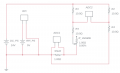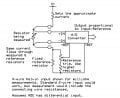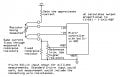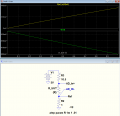# Resistance (current) sensing circuit using ADC

#### Multiplio

Joined Aug 9, 2022
6
Hi all,

This is my first post so apologies if I'm posting in the wrong place.

My aim is to measure the resistance between two terminals with one ADC across the terminals and one across a shunt resistor to measure current. The resistance is expected to vary between open circuit and hundreds of milli ohms, but I'm only interested in measuring detail in the first few ohms of resistance. I suspect the cables connecting the terminals to the device will also add a bit of resistance so the minimum resistance won't necessarily be zero, but I'm mainly interested in the change in resistance not the absolute value.

I have a 110 V DC power supply and will get a transformer to get a 5 V output for the ADCs and a Pi, and potentially another to lower the voltage in the testing circuit (arbitrarily picked as 24 V below).

However I'm not super confident on the circuit to connect the cables to the ADC, particularly with regard to maximising the voltage range of the output for the ADCs, and making sure the ground of the ADCs and the circuit are common.

I've attached my first attempt at a circuit using a wheatstone bridge, but would appreciate any suggestions/advice on whether it would work or whether something completely different would be better. The resistor values and 24 V supply for the testing circuit are temporary values as I'm not sure what would be most suitable in practice.Let me know if there's any more details I need to provide.

Thanks for the help!
Multiplio

#### crutschow

Joined Mar 14, 2008
32,889
My aim is to measure the resistance between two terminals
Are the terminals in a circuit, or is this an isolated resistance?

Is the variable resistance the one you want to measure?

#### KeithWalker

Joined Jul 10, 2017
2,872
It would be much simpler to supply a known constant current to the device under test and just use one ADC to measure the voltage across it.

•ErnieM

#### Multiplio

Joined Aug 9, 2022
6
Are the terminals in a circuit, or is this an isolated resistance?

Is the variable resistance the one you want to measure?
Sorry I should have specified - it's an isolated resistance (marked as variable on the diagram - probably could have done with a more descriptive name)

#### Multiplio

Joined Aug 9, 2022
6
It would be much simpler to supply a known constant current to the device under test and just use one ADC to measure the voltage across it.
Perhaps - how would you suggest creating the constant current supply? I've got plenty of theoretical experience, but given I've never tried building one in practice I thought I'd play it safe and stick to what I know I can do.

#### crutschow

Joined Mar 14, 2008
32,889

#### Multiplio

Joined Aug 9, 2022
6
What accuracy do you want?
+/- 5 milli ohms would be nice but if not, whatever I can get without adding too much complexity

#### crutschow

Joined Mar 14, 2008
32,889

#### Multiplio

Joined Aug 9, 2022
6
For what total resistance?
The minimum is 3.5 milli ohms but I'm not currently sure what the expected maximum is - I currently estimate a few hundred milli ohms.

Unfortunately I need to have the circuit finished before I'm able to physically test the resistance so it would be good to be able to change the circuit if the range turns out to be significantly different.

Thanks for your help with this!

#### Harry Trietley

Joined Jun 29, 2022
10
Here's a concept - not a full design - that needs only one ADC and NO constant current source. It takes advantage of the fact that analog to digital conversion is ratiometric - proportional to Input/Reference. If the input and reference increase or decrease by the same amount, the A/D result stays the same.

The first diagram uses an A/D converter with a differential input. The top resistor sets the approximate current. Whatever that current is, the same current flows through both the measured and reference resistors. If the measured resistor changes, or even if V+ changes, so will the current, but the ADC's result will still be proportional to Measured/Reference. I've played with my cheap DVM - it works that way. The measurement current changes when you change the measured resistance, but the result always is correct.

The second diagram is a variation on the theme, using a microcontroller or microprocessor instead of an ADC. It assumes the uC has a built-in ADC. Hopefully, the notes on the diagram are self-explanatory.

As noted on both, I've shown a Kelvin 4-wire input connection to minimize errors due to the connecting wire resistances. If the wire resistance errors are not significant, it works just as well with a standard 2-wire input. ￼•cmartinez and crutschow

#### ErnieM

Joined Apr 24, 2011
8,362
The low end of simple A2D's Ive seen are around 2 volts. Say the max resistance is 300 milli-ohms.

To get 2V on a .3 ohm resistor you need to drive it with 2V/.3ohms = 6.66 amps.

To use a more reasonable test current one would need an amplifier to boost the resistor's drop.

#### Harry Trietley

Joined Jun 29, 2022
10
The low end of simple A2D's Ive seen are around 2 volts. Say the max resistance is 300 milli-ohms.

To get 2V on a .3 ohm resistor you need to drive it with 2V/.3ohms = 6.66 amps.

To use a more reasonable test current one would need an amplifier to boost the resistor's drop.

Ernie, I think you are right. I didn't think that far when I wrote my ratiometric suggestion. The basic ratiometric idea still will work if you properly amplify the resistor's voltage but, as you say, doing a good amplifier (which would need to be differential) accurate to fractions of a millivolt is not quick and simple. (It surely could be done using a low-drift instrumentation amplifier IC. I wouldn't find it too difficult, but that's only because much of my experience is in signal conditioning and sensor electronics.)

Just for interest, I fired up my "cheap meter" again. Its lowest range is 200 ohms with 0.1 ohm resolution - no good for milliohms. Measuring 180 ohms, the voltage across the resistor was 0.301 V, implying 1.7 milliamps. With 0.1 ohms the voltage was about 0.25 mV (2.5 mA). I don't know whether that is amplified before it goes to the A/D because the IC chip is buried under a blob of epoxy.

#### crutschow

Joined Mar 14, 2008
32,889
to get 2V on a .3 ohm resistor you need to drive it with 2V/.3ohms = 6.66 amps.
True for a 2V full scale, but there are A/Ds that operate from a lower reference such as this MCP3301 with differential input, which can operate down to a 400mV reference (which equals the full scale conversion value).
That would require a current of 1.3A to get 400mV across 0.3Ω.
But since the TS only requested a ±5mΩ accuracy ( 1 out of 60), and that converter has 13-bit resolution, the test current and input voltage could be significantly reduced and still give the required accuracy.
For example, if a 1Ω value was set to 0.4V full scale (0.4A), than 1mΩ would still be 8 LSBs or 40 LSBs for 5mΩ.

That should avoid the need for an added amplifier.

Below is the simulation of the basic input for the post #10 circuit with a 1Ω maximum test resistance:
Note that the reference voltage (green trace) goes from 435mV to 400mV for a measurement resistance variation of 0 to 1Ω, while the AD differential input voltage (yellow trace) goes from 0 to 400mV, giving the measurement ratio.

For example at 0.5Ω, the AD simulated input voltage is 208mV and the Reference voltage is 416mV, showing the 0.5 ratio expected for that resistance.•Harry Trietley